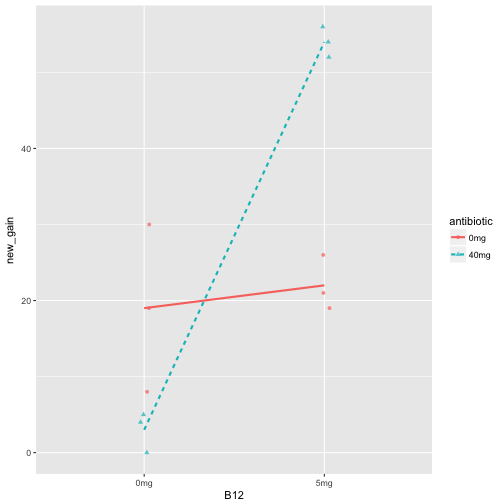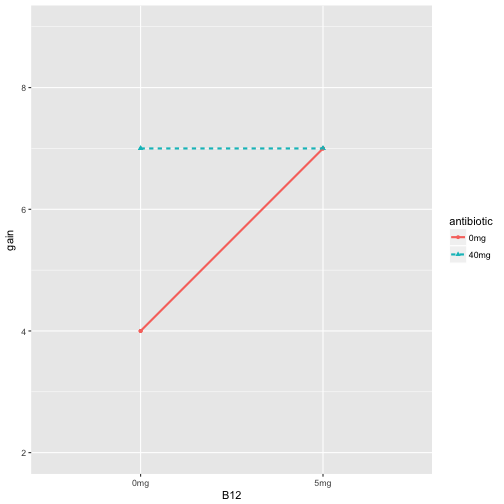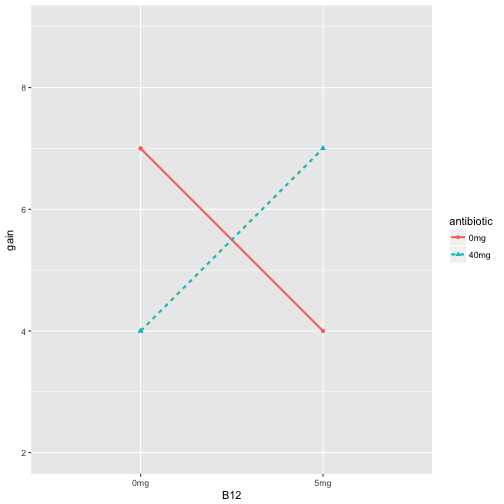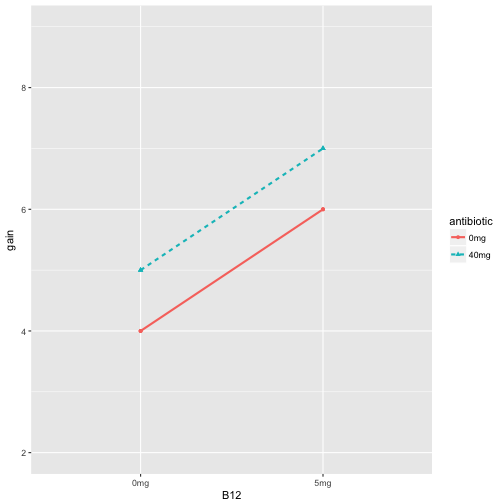# Review for Exam 2

Prof Randi Garcia
April 18, 2018

### Announcements

• HW 9, due today at 11:55pm on Moodle
• Exam 2 passed out today
• Due TUESDAY April 24, at 5:45pm

### Agenda

• Exam review
• Practice recognizing designs
• Design questions
• Writing diagrams and summary tables
• Formal ANOVA tables
• Practice with interaction graphs

### Design Questions

1. How many structural factors are there? What might you call them?
2. How many levels does each factor have? What are they?
3. How are the factors related? (i.e., crossing, blocking)
4. What is the response variable? What's its level of measurement?
5. What are the experimental units?
6. What's the name of this design?

### Three Ways to Block

1. Sort units into similar groups
2. Subdivide larger chunks of material into sets of smaller peices
3. Reuse subjects or chunks of material in each of sveral times slots

### Split Plot Design

If you suspect a design in a split-plot design, you should be able to answer the following questions:

1. What are the whole plots, that is, what is the nuisance factor?
2. What is the between-blocks factor? Is it observational or experimental?
3. What is the within-blocks factor? Is it observational or experimental?

### Example

In a study designed to shed light on the processes underlying facial recognition, Murray, Yong, and Rhodes (2000) presented each of 24 subjects with photos of several faces. There were three versions of each photo—an unaltered version, a distortion in which eyes and mouth were inverted, and a distortion in which the pupils of the eye were whitened and the teeth blackened. Each subject viewed each face for 3 seconds in 1 of 7 positions, from upright to upside down. Subjects were then asked to rate each face for bizarreness, with normal being 1 and very bizarre being 7. All subjects saw faces with all types of distortion and orientations.

### Example

In a probability-learning experiment conducted by Myers et al. (1983), each of 48 participants studies one of three texts (standard text, low explanatory text, and high explanatory text) that presented elementary probability concepts such as the addition and multiplication rules, and conditional and joint probability. The participants were tested on 6 story and 6 formula problems immediately following study, and they were tested on a second set of 12 problems two days later. Half of each group of participants received the story problems first, and the other half received the formula problems first (order constant across the two test days). The response variable is the proportion correct out of 12.

### Diabetic Dogs

The disease diabetes affects the rate of turnover of lactic acid in a system of biochemical reactions called the Cori cycle. This experiment compares two methods of using radioactive carbon-14 to measure rate of turnover. Method 1 is injection all at once, and method 2 is infused continuously. 10 dogs were sorted into two groups, 5 were controls and 5 had their pancreas removed (to make it diabetic). The rate of turnover was then measured twice for each dog, once for each method. The order of the two methods was randomly assigned.

### Diabetic Dogs

Split Plot/Repeated Measures Design, SP/RM[1,1]

### Plants in cups

The purpose of this experiment was to study the way one species of crabgrass competed with itself and with another species for nitrogen (N), phosphorus (P), and potassium (K). Bunches of crabgrass were planted in vermiculite, in 16 Styrofoam cups; after the seeds head sprouted, the plants were thinned to 20 plants per cup. Each of the 16 cups were randomly assigned to get one of 8 nutrient combinations added to its vermiculite. For example, yes-nitrogen/no-phosphorus/yes-potassium. The response is mean dry weight per plant, in milligrams.

### Plants in cups

Three-Way Basic Factorial Design BF

• aka, Completely Randomized Factorial Design CR

### Worms

Worms that live at the mouth of a river must deal with varying concentrations of salt. Osomoregulating worms are able to maintain relatively constant concentration of salt in the body. An experiment wanted to test the effects of mixtures of salt water on two species of worms: Nereis virens (N) and Goldfingia gouldii (G). Eighteen worms of each species were weighted, then randomly assigned in equal numbers to one of three conditions. Six worms of each kind were placed in 100% sea water, 67% sea water, or 33% sea water. The worms were then weighted after 30, 60, and 90 minutes, then placed in 100% sea water and weighted one last time 30 minutes later. The response was body weight as percentage of initial body weight.

### Worms

Split Plot/Repeated Measures Design, SP/RM[2,1]

### Schizophrenia

People with severe psychiatric problems find it harder to focus their attention; this theory predicts that psychiatrically normal subjects would be less affected than others by distracting sounds while they tried to remember things. In an experiment, participants were classified as 1) schizophrenic, 2) schizotypal, 3) borderline, or 4) psychiatrically normal. In each of a series of trials, subjects heard a voice read a series of digits and were asked to repeat them back. The digit strings were either 3, 4, or 7 digits long, and the trials either had a distracting male voice between the female voice reading the letters or it did not have interference. One response was defined by lumping together all the trials of a given type, such as four digits/no interference, and adding up the number of time the subject remembered the string correctly.

### Schizophrenia

Split Plot/Repeated Measures Design, SP/RM[1,2]

The Canada goose is a magnificent bird, but it can be a nuisance in urban areas in large numbers. One method of population control is to addle eggs in nests, but this method can hard adult females. Would removal of the eggs at the usual hatch date prevent harm? It is suspected that females nesting together at different sites are similar to each other. We randomly select 5 different sites, and we then randomly assign 5 nests per site to the addle with no removal condition, and 5 nests per site to the addle plus removal condition. The females at the nests are banded such that survival age can be measured later.

One-Way Complete Block Design CB

### Paper Helicopters

Paper helicopters can be cut from one half of an 8.5 by 11 sheet of paper. We can conduct an experiment by dropping helicopters from a fixed height and clocking the time it takes to drop. We can vary wing length: 4.25 in, 4.0 in, 3.75 in, and 3.5 in, as well as body width: 3.25 in, 3.75 in, 4.0 in, and 4.25 in. We'll make 32 planes and randomly assign them to the 16 combinations.

### Paper Helicopters

Two-Way Basic Factorial Design, BF

### Parks and Recreation

Deputy director of the Pawnee Parks and Rec department, Leslie Knope, needs to know how resistant different vegetative types are to trampling so that the number of visitors can be controlled in sensitive areas. Twenty lanes of a park are established, each .5 m wide and 1.5 m long. These twenty lanes are randomly assigned to five treatments: 0, 25, 75, 200, or 500 walking passes. Each pass consists of a 70-kg individual wearing boots, walking in a natural gait. One year after trampling, the average height of the vegetation along the lanes are measured.

### Parks and Recreation

Randomized Basic Factorial Design BF

### Albino Rats

Male albino laboratory rats are used routinely in many kinds of experiments. This experiment was designed to determine the requirements for protein and animo acid threonine in their food. Specifically, the experiment is interested in testing the combinations of eight levels of threonine (.2 through .9% of diet) and five levels of protein (8.68, 12, 15, 18, and 21% of diet). Baby rats were separated into five groups of 40 to form groups of approximately the same weight. The 40 rats in each group were randomly assigned to each of the 40 conditions. Body weight and food consumption were measured twice weekly, and the average daily weight gain over 21 days was recorded.

### Albino Rats

Two-Way Complete Block Design CB

### Drug Delivery

This experiment is interested in the blood concentration of a drug after it has been administered. The concentration will start at zero, then go up, and back down as it is metabolized. This curve may differ depending on the form of the drug (a solution, a tablet, or a capsule). We will use three subjects, and each subject will be given the drug three times, once for each method. The area under the time-concentration curve is recorded for each subject after each method of drug delivery.

### Drug Delivery

One-Way Complete Block Design CB

### Leafhoppers

An experiment was conducted to compare the effects of four sugar diets on the survival of leafhoppers. The four diets were glucose and fructose (6-carbon atoms), sucrose (12-carbon), and a control (2% agar). The experimenter prepared two dishes with each diet, divided the leafhoppers into eight groups of equal size, and then randomly assigned them to dishes. Then she counted the number of days until half the insects had died.

### Leafhoppers

Randomized Basic Factorial Design BF

### Gender Bias in STEM

In a randomized double-blind study (n = 127), science faculty from research-intensive universities rated the application materials of a student who was randomly assigned either a male or female name for a laboratory manager position. Faculty participants rated the male applicant as significantly more competent and hireable than the (identical) female applicant. These participants also selected a higher starting salary and offered more career mentoring to the male applicant. See materials here

### Gender Bias in STEM

Randomized Basic Factorial Design BF
- or SP/RM[1,1], depending on how you treat the response

### Acid Rain

“Clean” precipitation has a pH in the 5.0 to 5.5 range, but observed precipitation pH in northern New Hampshire is often in the 3.0 to 4.0 range. Is this acid rain hurting trees? 240 six-week-old yellow birch seedlings were randomly assigned to one of 5 groups. Each group received an acid rain mist at the following pH levels: 4.7, 4.0, 3.3, 3.0, and 2.3. After 17 weeks, the seedling were weight, and their total plant (dry) weight was recorded.

### Acid Rain

Randomized Basic Factorial Design BF

### Inappropriate Insects

Modern zoos try to reproduce natural habitats in their exhibits as much as possible. They try to use appropriate plants, but these plants can be infested with inappropriate insects. Cycads (plants that look vaguely like palms) can be infected with mealybugs, and the zoo wishes to test three treatments: 1) water, 2) horticultural oil, and 3) fungal spores in water. Five infested cycads are taken to to the testing area. Three branches are randomly selected from each tree, and 3 cm by 3 cm patches are marked on each branch. The number of mealybugs on the patch is counted. The three treatments then get randomly assigned to the three branches for each tree. After three days the mealybugs are counted again. The change in number of mealybugs is computed ($$before-after$$).

### Inappropriate Insects

One-Way Complete Block Design CB

Most people believe that country air is better to breather than city air, but how would you test it? You might start by choosing a response that narrows down what you mean by “better.” One feature of healthy lungs is tracheobronchial clearance—how fast they get rid of nasty stuff. To test this idea, investigators found 7 sets of mono-zygotic twins where one was living in the country and one in the city. Each person inhaled an aerosol of radioactive Teflon particles. Then the level of radioactivity was measured once right after inhaling, and again an hour later. The percent of the original radioactivity remaining was calculated.

One-Way Complete Block Design CB

Objectification theory (Fredrickson & Roberts, 1997) posits that American culture socializes women to adopt observers' perspectives on their physical selves. This self-objectification is hypothesized to (a) produce body shame, which in turn leads to restrained eating, and (b) consume attentional resources, which is manifested in diminished mental performance. An experiment manipulated self-objectification by having participants try on a swimsuit or a sweater. Further, it tested 21 women and 20 men, in each condition, and found that these effects on body shame and restrained eating replicated for women only. Additionally, self-objectification diminished math performance for women only.

Two-Way Basic Factorial Design, BF

### Creepy Animals

The effects of exposure to images of different domestic animal species in either aggressive or submissive postures on 4 different emotions was tested with a split-plot/repeated measures design. Using a computer to randomize, participants were randomly assigned to either view images of dogs or images of cats. All participants saw both an aggressive animal and a submissive animal, and their moods were assessed via self-report after each image. The order of presentation (aggressive then submission, or submissive then aggressive) was randomized to control for order effects.

### Creepy Animals

Split Plot/Repeated Measures Design, SP/RM[2,2]

### ANOVA Source Table for BF

• Using algebraic Notation

${y}_{ij}=\mu+{\alpha}_{i}+{e}_{ij}$

${H}_{0}:{\alpha}_{1}={\alpha}_{2}=...={\alpha}_{a}$

Source SS df MS F
Treatment $$n\sum_{i=1}^{a}(\bar{y}_{i.}-\bar{y}_{..})^{2}$$ $$a-1$$ $$\frac{{SS}_{Treatments}}{{df}_{Treatments}}$$ $$\frac{{MS}_{Treatments}}{{MS}_{E}}$$
Error $$\sum_{i=1}^{a}\sum_{j=1}^{n}({y}_{ij}-\bar{y}_{i.})^{2}$$ $$N-a$$ $$\frac{{SS}_{E}}{{df}_{E}}$$

### ANOVA Source Table for BF

${y}_{ijk}={\mu}+{\alpha}_{i}+{\beta}_{j}+{\alpha\beta}_{ij}+{e}_{ijk}$

Source SS df MS F
Treatment A $$\sum_{i=1}^{a}bn(\bar{y}_{i..}-\bar{y}_{…})^{2}$$ $$a-1$$ $$\frac{{SS}_{A}}{{df}_{A}}$$ $$\frac{{MS}_{A}}{{MS}_{E}}$$
Treatment B $$\sum_{j=1}^{b}an(\bar{y}_{.j.}-\bar{y}_{…})^{2}$$ $$b-1$$ $$\frac{{SS}_{B}}{{df}_{B}}$$ $$\frac{{MS}_{B}}{{MS}_{E}}$$
Interaction AB $$n\sum_{i=1}^{a}\sum_{j=1}^{b}(\bar{y}_{ij.}-\bar{y}_{i..}-\bar{y}_{.j.}+\bar{y}_{…})^{2}$$ $$(a-1)(b-1)$$ $$\frac{{SS}_{AB}}{{df}_{AB}}$$ $$\frac{{MS}_{AB}}{{MS}_{E}}$$
Error $$\sum_{i=1}^{a}\sum_{j=1}^{b}\sum_{k=1}^{n}({y}_{ijk}-\bar{y}_{ij.})^{2}$$ $$ab(n-1)$$ $$\frac{{SS}_{E}}{{df}_{E}}$$

### Formal ANOVA for CB

${y}_{ijk}={\mu}+{\tau}_{i}+{\beta}_{j}+{e}_{ijk}$

Source SS df MS F
Treatment $$\sum_{i=1}^{a}b(\bar{y}_{i.}-\bar{y}_{..})^{2}$$ $$a-1$$ $$\frac{{SS}_{T}}{{df}_{T}}$$ $$\frac{{MS}_{T}}{{MS}_{E}}$$
Blocks $$\sum_{j=1}^{b}a(\bar{y}_{.j}-\bar{y}_{..})^{2}$$ $$b-1$$ $$\frac{{SS}_{B}}{{df}_{B}}$$ $$\frac{{MS}_{B}}{{MS}_{E}}$$
Error $$\sum_{i=1}^{a}\sum_{j=1}^{b}({y}_{ij}-\bar{y}_{i.}-\bar{y}_{.j}+\bar{y}_{..})^{2}$$ $$(a-1)(b-1)$$ $$\frac{{SS}_{E}}{{df}_{E}}$$

### Formal ANOVA for the Latin Square

${y}_{ijk}={\mu}+{\alpha}_{i}+{\beta}_{j}+{\tau}_{k}+{e}_{ijk}$

• $${\mu}$$ is the benchmark
• $${\alpha}_{i}$$ is the row effect
• $${\beta}_{j}$$ is the column effect
• $${\tau}_{k}$$ is the treatment effect
• There are p rows, columns, and treatments

### Formal ANOVA for the Latin Square

${y}_{ijk}={\mu}+{\alpha}_{i}+{\beta}_{j}+{\tau}_{k}+{e}_{ijk}$

Source SS df MS F
rows $$\sum_{i=1}^{p}p(\bar{y}_{i..}-\bar{y}_{...})^{2}$$ $$p-1$$ $$\frac{{SS}_{A}}{{df}_{A}}$$ $$\frac{{MS}_{A}}{{MS}_{E}}$$
columns $$\sum_{j=1}^{p}p(\bar{y}_{.j.}-\bar{y}_{...})^{2}$$ $$p-1$$ $$\frac{{SS}_{B}}{{df}_{B}}$$ $$\frac{{MS}_{B}}{{MS}_{E}}$$
treatment $$\sum_{k=1}^{p}p(\bar{y}_{..k}-\bar{y}_{...})^{2}$$ $$p-1$$ $$\frac{{SS}_{T}}{{df}_{T}}$$ $$\frac{{MS}_{T}}{{MS}_{E}}$$
Error $$\sum_{i=1}^{p}\sum_{j=1}^{p}({y}_{ijk}-\bar{y}_{i..}-\bar{y}_{.j.}-\bar{y}_{..k}+2\bar{y}_{..})^{2}$$ $$(p-1)(p-2)$$ $$\frac{{SS}_{E}}{{df}_{E}}$$

### Algebraic Notation for the Spilt Plot Design

${y}_{ijk}={\mu}+{\alpha}_{i}+{\beta}_{j(i)}+{\gamma}_{k}+({\alpha\gamma})_{ik}+{e}_{ijk}$

• $${\mu}$$ is the benchmark
• $${\alpha}_{i}$$ effect of level i of the between-blocks factor, $$i$$ from $$1$$ to $$a$$
• $${\beta}_{j(i)}$$ effect of block $$j$$ (for level $$i$$ of the within block factor), $$j$$ from $$1$$ to $$n$$
• $${\gamma}_{k}$$ effect of level $$k$$ of the within-block factor, $$k$$ from $$1$$ to $$t$$
• $$({\alpha\gamma})_{ik}$$ interaction effect for level $$i$$ of the between-blocks factor with level $$k$$ of the within-blocks factor

### Formal ANOVA for the Spilt Plot Design

${y}_{ijk}={\mu}+{\alpha}_{i}+{\beta}_{j(i)}+{\gamma}_{k}+({\alpha\gamma})_{ik}+{e}_{ijk}$

Source SS df MS F
Between $$\sum_{i=1}^{a}tn(\bar{y}_{i..}-\bar{y}_{...})^{2}$$ $$a-1$$ $$\frac{{SS}_{A}}{{df}_{A}}$$ $$\frac{{MS}_{A}}{{MS}_{B}}$$
Blocks $$t\sum_{i=1}^{a}\sum_{j=1}^{n}(\bar{y}_{ij.}-\bar{y}_{i..})^{2}$$ $$N-a$$ $$\frac{{SS}_{B}}{{df}_{B}}$$ $$\frac{{MS}_{B}}{{MS}_{E}}$$
Within $$\sum_{k=1}^{t}N(\bar{y}_{..k}-\bar{y}_{...})^{2}$$ $$t-1$$ $$\frac{{SS}_{T}}{{df}_{T}}$$ $$\frac{{MS}_{T}}{{MS}_{E}}$$
Interaction $$\sum_{i=1}^{a}\sum_{k=1}^{t}n(\bar{y}_{i.k}-\bar{y}_{i..}-\bar{y}_{..k}+\bar{y}_{...})^{2}$$ $$(a-1)(t-1)$$ $$\frac{{SS}_{AT}}{{df}_{AT}}$$ $$\frac{{MS}_{AT}}{{MS}_{E}}$$
Error $$\sum_{i=1}^{a}\sum_{j=1}^{n}\sum_{k=1}^{t}({y}_{ijk}-\bar{y}_{i.k}-\bar{y}_{ij.}+\bar{y}_{i..})^{2}$$ $$(N-a)(t-1)$$ $$\frac{{SS}_{E}}{{df}_{E}}$$

### Research Questions in a BF Design

1. Does treatment A have an effect on the response variable?
• Is there a main effect of factor 1?
2. Does treatment A have an effect on the response variable?
• Is there a main effect of factor 2?
3. Does being in a specific combination of treatments have an effect over and above the additive effects of treatment A and B alone?
• Is there an interaction between factor 1 and factor two?

### Interaction Mantra

An interaction is present if the effect of one factor is different across levels of the other factor.### Interaction Graphs

For each of the following interaction graphs, answer the following questions with YES or NO.

1. Is there a main effect of B12?
2. Is there a main effect of antibiotics?
3. Is there an interaction between B12 and antibiotics?

### Practice 1### Practice 11. YES
2. YES
3. YES

### Practice 2### Practice 21. NO
2. NO
3. YES

### Practice 3